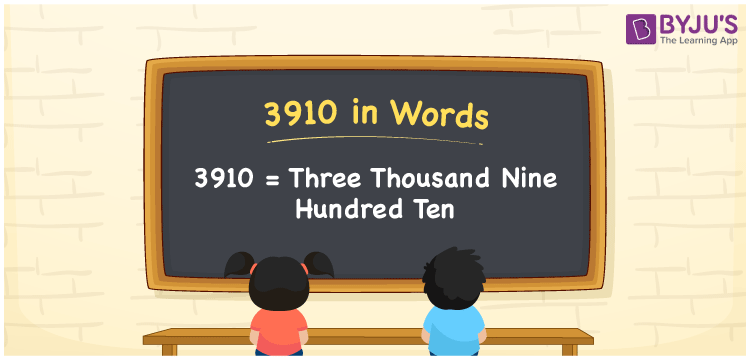# 3910 in Words

3910 in words is written as Three thousand nine hundred ten. In both the International System of Numerals and the Indian System of Numerals, 3910 is written as Three thousand nine hundred ten. The number 3910 is a Cardinal Number as it represents some quantity. For example, “that item costs 3910 rupees”.

 3910 in Words Three thousand nine hundred ten Three thousand nine hundred ten in Number 3910

## 3910 in English Words

3910 in English words is read as “Three thousand nine hundred ten”.## How to Write 3910 in Words?

To write 3910 in words, we shall use the place value chart. In the place value chart, put 3 in the thousands, 9 in the hundreds, 1 in the tens, and 0 in the ones, respectively. Let us make a place value chart to write the number 3910 in words.

 Thousands Hundreds Tens Ones 3 9 1 0

Thus, we can write the expanded form as

3 × Thousand + 9 × Hundred + 1 × Ten + 0 × One

= 3 × 1000 + 9 × 100 + 1 × 10 + 0 × 1

= 3000 + 900 + 10 + 0

= 3910

= Three thousand nine hundred ten.

3910 is a natural number, the successor of 3909 and the predecessor of 3911.

3910 in words – Three thousand nine hundred ten

• Is 3910 an odd number? – No
• Is 3910 an even number? – Yes
• Is 3910 a perfect square number? – No
• Is 3910 a perfect cube number? – No
• Is 3910 a prime number? – No
• Is 3910 a composite number? – Yes

## Frequently Asked Questions on 3910 in Words

Q1

### How to write 3910 in words?

3910 in words is written as Three thousand nine hundred ten.
Q2

### How to write 3910 in the International and Indian System of Numerals?

In both, the system of numerals, 3910 in words, is written as Three thousand nine hundred ten.
Q3

### How to write 3910 in a place value chart?

In the place value chart, write 3 in the thousands, 9 in the hundreds, 1 in the tens, and 0 in the ones, respectively.